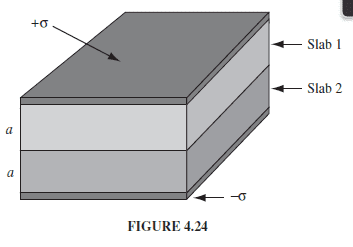# Electrical Potential and an Integration path

I have been looking everywhere for a clear explanation for the following: How do I know which way to integrate the electrical field, when I'm looking for the potential between to points?

Example:I have to find the potential between the top conducting plate and the bottom conducting plate. Assume that i got an expression for the field in both i linear dielectric slabs. My book says:

$$V = \int_\mathcal{O}^r \vec{E} \cdot d\vec{l}$$

However how do I translate that. Lets forget anything about the actually expression for ##\vec E##. Obviously we are going from ##0## til ##2*a## or the other way around. What dictates the path? I know what the answer to the assignment I'm doing is, but I do not see why my result (which is the same but negative) is wrong. It has something to do with the minus in front and the reference points. Can someone please clarify, because this has been driving me nuts for weeks now.

(The right answer comes from integrating from ##0## to ##2*a##)

#### Attachments

Homework Helper
Gold Member
I have been looking everywhere for a clear explanation for the following: How do I know which way to integrate the electrical field, when I'm looking for the potential between to points?

Example:
View attachment 231793

I have to find the potential between the top conducting plate and the bottom conducting plate. Assume that i got an expression for the field in both i linear dielectric slabs. My book says:

$$V = \int_\mathcal{O}^r \vec{E} \cdot d\vec{l}$$

However how do I translate that. Lets forget anything about the actually expression for ##\vec E##. Obviously we are going from ##0## til ##2*a## or the other way around. What dictates the path? I know what the answer to the assignment I'm doing is, but I do not see why my result (which is the same but negative) is wrong. It has something to do with the minus in front and the reference points. Can someone please clarify, because this has been driving me nuts for weeks now.

(The right answer comes from integrating from ##0## to ##2*a##)
For an EMF with an electric field ## \vec{E} ##, the voltage ## V=+\int \vec{E}_{induced} \cdot dl ##. For an electrostatic potential ## V=-\int \vec{E} \cdot dl ##. ## \\ ## The reason for this is arguably that in the case of an electric field from an EMF in a conductor, there is necessarily an electrostatic field that gets generated that is equal and opposite the Faraday electric field, in order to keep the current from becoming infinite. Instead, the total electric field must nearly vanish in the conductor.

For an EMF with an electric field ## \vec{E} ##, the voltage ## V=+\int \vec{E}_{induced} \cdot dl ##. For an electrostatic potential ## V=-\int \vec{E} \cdot dl ##. ## \\ ## The reason for this is arguably that in the case of an electric field from an EMF in a conductor, there is necessarily an electrostatic field that gets generated that is equal and opposite the Faraday electric field, in order to keep the current from becoming infinite. Instead, the total electric field must nearly vanish in the conductor.

I hate to so publicly display my plebian nature, but I have absolutely no idea what you mean.

EDIT: I have read what you wrote a couple of times know. I'm embarrassed to admit I don't even know what questions to ask either.

Homework Helper
Gold Member
I hate to so publicly display my plebian nature, but I have absolutely no idea what you mean.
EMF ## \mathcal{E}=-\frac{d \Phi}{dt} ## is known as an induced/Faraday EMF, where voltage ## V= \mathcal{E}=+\int \vec{E}_{induced} \cdot dl ##.## \\ ## (If you compute the voltage coming from an inductor, getting the correct sign can be important to have the calculations be completely consistent). ## \\## For the electrostatic potential ## V=-\int \vec{E} \cdot dl ##, which is also a voltage, the electric field integral gets a minus sign.

EMF ## \mathcal{E}=-\frac{d \Phi}{dt} ## is known as an induced/Faraday EMF, where ## \mathcal{E}=+\int \vec{E}_{induced} \cdot dl ##. ## \\## For the electrostatic potential ## V=-\int \vec{E} \cdot dl ##, the voltage gets a minus sign.

I have never heard of EMF nor seen the equations you just wrote before. The expressions of the fields I use in the actual assignment, is found from the displacement field equation. Is that related?

Homework Helper
Gold Member
I have never heard of EMF nor seen the equations you just wrote before. The expressions of the fields I use in the actual assignment, is found from the displacement field equation. Is that related?
You need to show more detail, but then, it really belongs as a homework problem in the homework section. ## \\ ## I'm surprised you are already seeing a capacitor with dielectric layers, but they have yet to teach you about the Faraday EMF, which is caused by changing magnetic fields, in the physics class that you have.

You need to show more detail, but then, it really belongs as a homework problem in the homework section. ## \\ ## I'm surprised you are already seeing a capacitor with dielectric layers, but they have yet to teach you about the Faraday EMF, which is caused by changing magnetic fields, in the physics class that you have.

We are using Griffith 4. Edition. Dielectrics is the last part of electrostatics, before magnetism is introduced. And yea I'd didn't really want help with the assignment itself as much as figure out what I'm missing about the potential.

vanhees71
$$\vec{\nabla} \cdot \vec{D}=\rho, \quad \vec{\nabla} \times \vec{E}=0, \quad \vec{D}=\epsilon \vec{E},$$